Volume 13 Supplement 13

# Model averaging strategies for structure learning in Bayesian networks with limited data

## Abstract

### Background

Considerable progress has been made on algorithms for learning the structure of Bayesian networks from data. Model averaging by using bootstrap replicates with feature selection by thresholding is a widely used solution for learning features with high confidence. Yet, in the context of limited data many questions remain unanswered. What scoring functions are most effective for model averaging? Does the bias arising from the discreteness of the bootstrap significantly affect learning performance? Is it better to pick the single best network or to average multiple networks learnt from each bootstrap resample? How should thresholds for learning statistically significant features be selected?

### Results

The best scoring functions are Dirichlet Prior Scoring Metric with small λ and the Bayesian Dirichlet metric. Correcting the bias arising from the discreteness of the bootstrap worsens learning performance. It is better to pick the single best network learnt from each bootstrap resample. We describe a permutation based method for determining significance thresholds for feature selection in bagged models. We show that in contexts with limited data, Bayesian bagging using the Dirichlet Prior Scoring Metric (DPSM) is the most effective learning strategy, and that modifying the scoring function to penalize complex networks hampers model averaging. We establish these results using a systematic study of two well-known benchmarks, specifically ALARM and INSURANCE. We also apply our network construction method to gene expression data from the Cancer Genome Atlas Glioblastoma multiforme dataset and show that survival is related to clinical covariates age and gender and clusters for interferon induced genes and growth inhibition genes.

### Conclusions

For small data sets, our approach performs significantly better than previously published methods.

## Introduction

In the last ten years there has been a great deal of research published on learning Bayesian networks from data . Most of the work in structure discovery in Bayesian networks has focused on designing computationally tractable procedures for searching the space of networks, and on devising metrics for scoring networks during search. However, the problem of determining the quality and robustness of learned structures in the context of limited data remains largely open. Structure learning algorithms are known to be unstable — small changes in training data can cause large changes in the learned structures. As Bayesian network learning begins to find serious application in biology , there is an increasing need for learning protocols that can not only discover network features, such as edges and Markov blankets, from small amounts of data, but can also determine an accurate confidence estimate for those features. In many biological and clinical settings, the size of the dataset may be severely limited, either by high cost or by the limited number of cases from which data can be collected (for instance, people affected by a rare disease) . In such settings, it is worthwhile to use substantial amounts of computation in order to achieve as accurate a result as possible. Model averaging using bootstrap replicates (that is, bagging) with feature selection by thresholding  is a widely used solution . Unfortunately, there is no established method for determining feature-selection thresholds. There is no prescription for the choice of bootstrap technique: the ordinary non-parametric bootstrap , a bias-corrected version of the non-parametric bootstrap , or the more continuous Bayesian bootstrap . Whether the choice of bootstrap method interacts with the choice of scoring function is unknown, as is the number of bootstrap replicates needed to achieve a certain level of confidence in the final model.

In this paper, our goal is to understand the interactions between choice of bootstrap method and scoring function, size of training data and number of bootstrap replicates as well as the choice of feature-selection threshold on averaged models. Unlike linear regression, for which closed form expressions for the performance of model-averaging procedures can be derived , our model class is too complex to permit the analytical derivation of quality guarantees. We therefore use experiments on two moderately sized Bayesian networks with known structure (ALARM and INSURANCE) to systematically study the effects of varying the bootstrap technique, the amount of training data, the number of bootstrap replicates, as well as the scoring function for network learning. We design a variant of permutation testing to automatically select thresholds for feature selection in bootstrap averaged (bagged) models and construct a loose upper bound for the number of bootstrap replicates needed for stable estimates of feature probabilities.

The variance in the structure of learned networks comes in part from the data, which is usually a single realization from some unknown true model. This is schematically depicted in Figure 1, where possible data sets sampled from the true model lie on the x axis, and potential Bayesian networks on the given set of variables are on the y axis. The contours denote regions in which the posterior probability of the network given the data are the same. Given a single data set, exploration in the structure space is completely determined by the parameters of the search algorithm. This is denoted by the vertical dotted blue line in Figure 1.

When the amount of data is small relative to the size of the model, the posterior probability of the model given the data is not sharply peaked around a single model . To correctly assess the probability of a structural feature (for instance, an edge) in the model, we need to average over the entire ensemble of networks with similar posterior probabilities. If it is reasonable to assume that all high-scoring structures are equally good, we have the probability of a feature e given data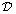to be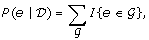(1)

or we can compute Bayesian posterior probabilities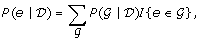(2)

where we weight each feature in proportion to the posterior probability of the structure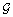in which it occurs.

For large networks, the direct estimation of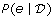is intractable. Structure learning algorithms approximate the computation in Equations 1 or 2 by only considering high scoring structures encountered during search. These structures tend to be quite similar to one another, as evidenced by the fact that feature (such as, edge) probabilities computed from them are usually close to 0 or 1. Computing feature probabilities by MCMC sampling over structures drawn from the posterior distribution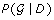has been proposed by Madigan and York  and refined by Giudici et al. . These techniques do not scale to large networks, and have been shown to be slow-mixing .

A different approach to estimating feature probabilities is to sample in different sections of the structure space by perturbing the data set. In effect, we follow the horizontal green dotted line parallel to the x axis in Figure 1. By taking bootstrap resamples from the original dataset, learning a single high-scoring Bayesian network from each resample, and averaging feature probabilities over these networks, we can obtain feature probability estimates from a more diverse population of structures. This is the motivation behind Algorithm 1 shown below, proposed in . A final network is assembled by including only those structural features that have estimated probabilities above a pre-specified threshold t, which is given as an input to the algorithm.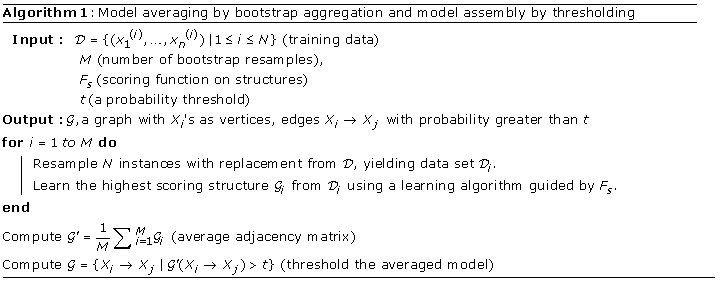The practical application of this algorithm, particularly in the context of limited data requires answers to many questions. Specifically:

• How many bootstrap resamples M are required to obtain stable estimates of the feature probabilities in bagged models?

• Which scoring functions F s work best with bagging? Friedman et al.  only used BDe as a scoring function in their bootstrap experiments. Hartemink et al.  offer experimental evidence that that BDe outperforms MDL/BIC in a bagging context. It is an open question how scoring functions such as DPSM compare to BDe.

• Is the bias in bagged Bayesian networks arising from the discreteness of the bootstrap method  a significant problem? If so, would a continuous version of bootstrap, like the Bayesian bootstrap  be a better choice for bootstrap resampling than the ordinary discrete bootstrap?

• How do we select thresholds for learning statistically significant features over averaged models? Does the choice of threshold depend on the scoring function, problem domain, sample size, and bootstrap resampling technique?

• Should a single high scoring structure be learned from a bootstrap replicate (as shown in Algorithm 1), or an averaged ensemble of high scoring structures (double averaging, as shown in Figure 2)?

The paper is organized as follows. In the next section, we review the problem of learning the structure of Bayesian networks from data, and describe the state of the art in scoring functions, search algorithms, and bootstrapping techniques. In the following section, we present an extensive experimental study that explores the space of model-averaging strategies in the context of the ALARM network. We then describe our technique for automatically selecting a probability threshold for feature selection. Our threshold selection criterion is designed to minimize the number of false positive features in bagged models. We conclude with a characterization of an effective model-averaging strategy in contexts with limited data.

## Learning the structure of Bayesian networks

### Bayesian networks

Bayesian networks are a compact, graphical representation of multivariate joint probability distributions on a set of variables. The graph structure reflects conditional independence relationships between the random variables. Formally, a Bayesian network for a set X = {X1,…, X n } of n discrete random variables is a pair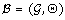whereis a directed acyclic graph whose vertices represent the random variables X1,… , X n , and whose edges represent direct dependencies between these variables. Θ represents the set of conditional probability distributions of the form ΘXi| U i = P(X i |U i ), 1 ≤ in, where U i denotes the parents of variable Xi in the graph. The joint probability distribution P(X1,…, X n ) encoded by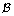can be reconstructed as the product of the individual conditional probability distributions in Θ: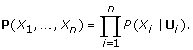### Learning Bayesian networks from data: scoring functions

The problem of learning a network from data is normally posed as an optimization problem: Given a set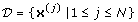of instances drawn from a multivariate joint probability distribution P(X), find a network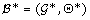which maximizes the posterior probability of the network given the data: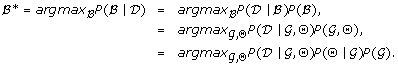The prior over network structures has two components: a discrete probability distribution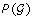over graph structures, and for each possible graph, a density measure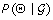over possible values of the parameter Θ. The simplest, and most common choice for both the priorover graphs as well as the parameter distribution, is the uniform distribution. Assuming that the data is sampled i.i.d., the likelihood of the data given a networkis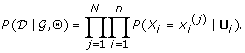The posterior probabilityis called a scoring metric or scoring function for Bayesian network learning algorithms. By marginalizing over all choices for the parameter distribution Θ, we can writeas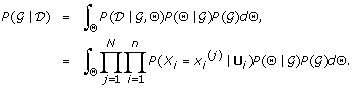The scoring functionsimplifies under the assumption of uniform structure and parameter priors to the following, called the K2 metric in , and the UPSM score in :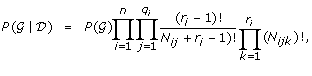where r i is the number of values that X i takes, and q i is the number of values that the parents of X i intake. N ijk is the number of instances inin which variable X i has its kth value, and its parents take their jth value, and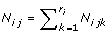.

A generalization of the above scoring metric uses Dirichlet priors (λ >= 1) for. The parameter priors for each variable are considered independent of one another, an assumption called global parameter independence in ,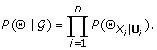In addition, the parameter modularity assumption is made . For two graph structuresand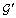such that the parents of node X i are the same in both graphs, we have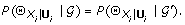The graph structure parameter prioris assumed to satisfy structural modularity; that is, it can be factored as a product of distributions over the possible parent sets of node X i in the graph. Under these assumptions, the posterior probability of the graph structurecan be written in fully factored form as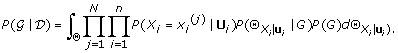The scoring function with these assumptions reduces to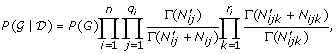where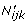is the Dirichlet distribution order for variable i with value k and parent value j, and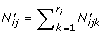. When the Dirichlet orders for all sets of parameters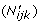are set to a constant λ, the score is called DPSM . When we assume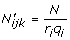, it is called the Bayesian Dirichlet (BDe) metric . The choice ofis critical, particularly for small data sets. If these parameters are large, makingdominate the N ijk values, the available data has less influence in determining the space of structures explored.

There is another family of scoring functions based on maximizing the likelihood function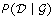. The first among these is the BIC score, also known as the Schwartz criterion, which is derived from the Laplace approximation to the logarithm of( , page 64) and defined by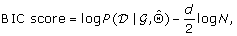where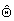is the maximum likelihood estimate of Θ givenand D, d is the number of free parameters in Θ, and N is the size of the training sample. The number of free parameters in Θ is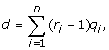where r i is the number of values that variable X i can take, and q i is the number of values that the parents of X i incan take. Direct maximization of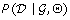yields overfitted models, because the likelihood of the data givenand Θ can be increased by increasing the complexity of(that is, by adding an edge). The second termcan be seen as a penalty term to compensate for model complexity. Finding a structurewith the highest BIC score is equivalent to finding a structure with the highest approximate posterior probability given the data D. As the sample size N → ∞, the probability that the BIC criterion selects the true model approaches 1. However, with finite sample sizes, BIC tends to select models that are much simpler than the true model, due to the penalty term.

The MDL, or the Minimum Length Description scoring function has its origins in data compression and information theory. The best model for a data set, according to the MDL, is one that minimizes the sum of the length (in bits) of the encoding of the model itself, and the length of the encoding of the data given the model. When applied to Bayesian network structure learning, the MDL score is the sum of the description length of the graph, the description length of the conditional probability parameters Θ given, and that of the datagivenand Θ. The description length of the graphis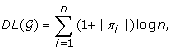where |π i | is the number of parents of X i in, and n is the number of variables X i in X. The description length of Θ is the product of the total number of independent parameters for specifying each conditional probability table, and the number of bits needed to encode each of these parameters for a data set of size N. Define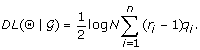Finally, the number of bits needed to encode the data given the model is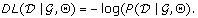Thus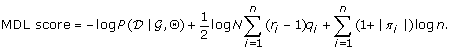Minimizing the MDL scoring function yields a model with the shortest description length. If we ignore the description length of the graph,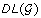, the MDL score equals the BIC score with reversed sign. The MDL scoring function favors graphs in which nodes have fewer parents, because it reduces the encoding length of Θ and.

To summarize, scoring functions belong to one of two families. Bayesian scoring functions, of which K2, DPSM, and BDe are examples, represent the posterior probabilityof a structuregiven data. The second family consists of complexity-penalized likelihood measures, of which BIC and MDL are examples. MDL can also be justified from an information-theoretic perspective. These scoring functions embody different assumptions about priors on the space of structures as well as priors on parameter distributions given the structures. There are few systematic studies in the literature to guide choice of scoring functions for specific applications. Below we show a comparative analysis of the performance of these scoring functions in the context of limited data.

### Learning Bayesian networks from data: local search algorithms

The problem of learning a network which maximizesis usually posed as a combinatorial optimization problem. It is known to be NP-complete , so local search algorithms guided by scoring functions are used to find approximate solutions.

The local search algorithm used in our experiments is greedy hill-climbing with randomized restarts and Friedman’s sparse candidate algorithm  with k = 6 (maximum number of parents per node). Every network explored by the algorithm during the search is recorded, and no network is ever considered twice. Initially, the k most likely parents for each node, the candidates, are selected by scoring all networks with a single edge between two nodes. Denote by K the set of all networks in which the parents of every node belong to the set of k candidate parents for that node. A list of starting networks containing all networks in K with up to two edges is then generated. A starting network is picked at random from this initial list. From this starting network, all neighboring networks in K that have not been considered before and which differ by an additional edge, one less edge, or a reversed edge are evaluated. The highest scoring neighboring network, if its score is higher, replaces the current network. The search is continued until no new networks are generated or all generated networks score less than the current network. New sets of k candidate parents are then generated following Friedman’s algorithm, and the search is continued. New candidate parents sets are picked until a previously seen set of candidate parents is revisited, or C = 10 different candidate parent sets have been considered. Such searches starting from a randomly picked member of the initial network list are performed a total of M1 = 25 times. Another M2 = 25 such searches are performed starting from a network chosen randomly from all of those seen during the first M1 searches. Network features are determined from the highest scoring network(s) visited over all searches.

### Bootstrap and Bayesian bootstrap

The bootstrap is a general tool for assessing statistical accuracy of models learned from data. Suppose we have a data set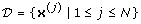, where each x(j) is a vector of size n drawn from the cross product of the domains of variables X1,…, X n . The basic idea is to randomly draw datasets with replacement from, with each sample the same size as the original set, that is, N. This is done B times, producing B bootstrap replicates, as shown on the left in Figure 2. We learn Bayesian networks from each bootstrap resample. We can then analyze the networks generated over the B resamples; for example, producing means and variances on frequencies of structural features.

Each example inis represented between 0 and B times among the bootstrap resamples. Thus one can can think of the standard bootstrap procedure as assigning each example inan integer weight drawn from a multinomial distribution, representing its number of occurrences in the B resamples. The probability of not including a specific example in a resample is about 1/e ≈ 37%. Since an example contributes to the count N ijk in the scoring function; dropping examples biases the counts, and the structures that are learned from them . Whether the discreteness of the bootstrap leads to the generation of more complex graphs as claimed in , with more false positive features, or whether it leads to structures with missing edges (false negative features) because of undersampling of weaker relationships in the data, is an open one.

An alternative approach is to use the Bayesian bootstrap . It is a Bayesian resampling procedure that is operationally similar to the ordinary non-parametric bootstrap. In the Bayesian bootstrap, examples are assigned continuously varying weights drawn from a Dirichlet distribution. Not surprisingly, the Bayesian bootstrap procedure has a Bayesian interpretation. Assume that examples are drawn from some unknown multivariate distribution P(X), and that we have no specific priors on that distribution. The uninformative prior on P combined with the multinomial sample likelihood yields, via Bayes Theorem, a Dirichlet posterior distribution on the fraction of the original population that each sampled example represents. The ensemble of Bayesian bootstrap resamples, and the distribution of statistics derived from them, can be viewed as samples from a Bayesian posterior distribution. The continuously varying weights of the Bayesian bootstrap ensure that there is a vanishingly small chance of assigning a zero weight to any example in a resample. Thus, all of the inter-relationships between examples are preserved in the resample, but in reweighted form. Statistics derived from these resamples do not embody the bias introduced by the discreteness of the regular bootstrap.

We now turn to the question of the number of bootstrap resamples needed to form accurate estimates of structure feature probabilities.

### Estimating a bound on the number of bootstrap resamples

The probability of a feature e from a bootstrap resample,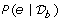, will be exactly 0 or 1 if estimated from the single best network. In double averaging, the, although estimated from multiple high-scoring networks, have highly bimodal distributions, each being either close to 0 or close to 1, with few exceptions. Consequently, we here approximate the feature probabilities from each resample as either 0 or 1. The averaged or bagged feature probabilities then have binomial distributions that in the large resample limit approximate a normal distribution with mean p and variance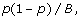(3)

where p is the probability of getting a probability value of 1 in each resample, and B is the number of resamples. For fixed number of resamples B, the largest variance is obtained when p = 0.5. The largest feature probability variance can therefore be estimated by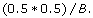(4)

For B = 200 (experimental setting used in  and suggested in ), the largest feature probability variance is 0.25/200 ≈ 0.00125 yielding a standard deviation of approximately 0.035. Three standard deviations around the mean of 0.5 yields a probability range of 0.4 – 0.6. To obtain a more reasonable standard deviation of about 0.01 on link probabilities, and hence a three-sigma range of 0.47-0.53, we need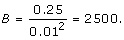In practice, we are most concerned about feature probabilities close to the cutoff threshold. For features probabilities p greater than 0.5, the number of resamples required to achieve a standard deviation of 0.01 is approximately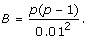Even for a large p, for instance 0.9, this works out to be 900 resamples.

In practice, our resample feature probabilities are sometimes not exactly 0 or 1, so we expect the above to be slight overestimates.

## ALARM network simulation study

To understand how different bagging methods, scoring metrics, and their parameters affect learning performance, we conducted an extensive simulation study using the well known ALARM network. This network contains 37 discrete nodes, with two to four values per node, and 46 edges. We drew a master dataset containing 100,000 data points from the ALARM network’s joint probability distribution.

To evaluate the effect of different strategies and parameters on learning performance, we extract a subset from that master dataset and apply the network learning strategy being tested. In all cases, the core learning strategy is the greedy hill-climbing algorithm with random restarts described above. We compute posterior probabilities of network features, such as edges, by determining how often each such feature occurs in the best single network learnt from each bootstrap resample. A slight variation is double averaging, which first computes feature probabilities for each resample by (Bayesian) averaging of multiple high-scoring networks learnt for that resample, and then averages these probabilities across resamples. In either case, the bagging process produces estimates of posterior probabilitiesfor edge features. Given a threshold 0 ≤ t ≤ 1, we will call an edge e present if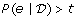. An edge is a true positive if it is present in the known ALARM network. An edge is labeled a false positive if its posterior probability exceeds t, and it is absent in the reference network. An edge is a true negative if its posterior probability is less than t and it is absent in the reference network. Edges with posterior probabilities less than t that are present in the reference network are false negatives. We do not consider edge direction in our assessments; an edge is defined to be present if the sum of probabilities of occurrence in both directions exceeds the threshold t. We report the number of false positives and false negatives as a function of the threshold t and use it to assess the quality of models learned. Unless otherwise stated, the learning performance we report are averages obtained by applying the above process to 60 independent subsets of the master dataset.

### Number of bootstrap resamples required

To estimate the number of bootstrap resamples required, we performed 60 independent Bayesian bootstrap bagging analyses of a single dataset containing 250 data points. Each analysis used identical data, but a different seed for the pseudo-random number generator. To illustrate the convergence of the different bootstrap runs, we choose the edge between PULMEMBOLUS and PAP as a representative example. Figure 3 shows the cumulative probability of this edge being present. Every independent bootstrap run is plotted as a faint grey line. Overlapping lines are darker in proportion to the number of lines involved. The solid blue line is the cumulative average across all bootstrap runs of this posterior probability. The solid red line (on the right-hand side axis) is the variance of the probability across the bootstrap runs. The dotted blue lines indicate three standard deviations (estimated across the bootstrap runs) above and below the sample mean. The dotted red line indicates the expected variance if we assume that the result for each bootstrap resample is either 0 or 1. The variance curve shows that to get a standard deviation of 0.03 to 0.01 for this edge (corresponding to variance of 10–3 to 10–4), we need between 500 and 2,500 resamples. Most experimental studies in the literature use 200 bootstrap samples, a figure suggested by . However, this experiment (as well as others we have done) indicates we need an order-of-magnitude more resamples for structure learning with limited data. For the remainder of this study, we use 2,500 bootstrap resamples in all experiments.

### Effect of bagging on learning performance

Figure 4 shows the impact of bagging on learning performance, for datasets with 250 data points. The scoring function used is DPSM with λ = 1. The false positive/false negative tradeoff curve with bagging lies well below the tradeoff curves without bagging. For a fixed number of false positives, bagging yields significantly fewer false negatives. Similarly, for a fixed number of false negatives, bagging yields fewer false positive edges. In the structure learning context, bagging enables us to estimate the posterior probabilities of edge features more accurately, with additional resamples resulting in substantial reduction in variance. This variance reduction translates to more accurate structures learned from the same data. Similar trends are observed for other scoring metrics as well as other bagging approaches.

### Effect of double bagging

The learning procedure, with its multiple restarts, often produces several top networks with very similar high scores. Since the greatest computational effort, by far, is the learning procedure, it might be possible to improve learning performance by deriving a composite probability for each feature from several of these top scoring networks from each resample. We compared bagging using only the best network, to bagging of feature probabilities estimated by a simple average and a Bayesian average of multiple, distinct high-scoring networks from each bootstrap resample. This averaging over the top networks occurs prior to bagging, as shown on the right hand side of Figure 2. Figure 5 shows the results of double averaging versus picking the single best on the ALARM data set with scoring function DPSM (λ = 1).

### Effect of bias-correction strategies on model accuracy

To determine the impact of bias introduced by discrete bootstrap resampling, and the effect of changes to the scoring function to account for it, we compared ordinary bagging, ordinary bagging with a bias corrected scoring function , and Bayesian bagging on the same ALARM data set with 250 points. The upper panel in figure 6 shows the false positive/false negative tradeoff curves for the three bagging approaches. It clearly shows that the bias corrected scoring metric performs worse than ordinary and Bayesian bagging. The lower panel in figure 6, which shows the number of structural errors (false positives and false negatives) as a function of threshold choice, reinforces this result. Similar trends are observed for different dataset sizes and different choices of scoring functions.

Below we offer a possible explanation for this result. Ordinary bagging and Bayesian bagging appear to have very similar performance, with Bayesian bagging outperforming ordinary bagging in terms of the number of structural errors for thresholds above 0.72. Since this threshold range is likely to be the range of greatest interest, especially in the context of limited data, we believe that Bayesian bagging is a better choice for structure learning of Bayesian networks.

### Effect of local scoring function

Figure 7 shows the impact of the scoring metric on learning performance, using Bayesian Bagging on datasets with 250 data points. Similar trends are observed for datasets of other sizes. Figure 7 makes it clear that MDL/BIC performs very poorly, and also confirms Hartemink’s result  that BDe performs better than MDL/BIC. Surprisingly, DPSM with λ = 1 performs the best. As the next section shows, DPSM with values of λ less than 1 perform even better. This surprising experimental result reveals an important requirement for scoring functions to be effective with bagging, particularly in contexts with limited data.

### Effect of λ for DPSM scoring function

Figure 8 shows the impact of λ on the performance of the DPSM scoring metric on ALARM. Learning performance, measured in terms of the false positive/false negative tradeoff curve continues to improve as λ is reduced until approximately 0.1 to 0.2, below which learning performance rapidly deteriorates. In subsequent experiments, we therefore use the DPSM metric with λ = 0.1.

As λ values are lowered, the edge density of the Bayesian averaged graphs produced from each bootstrap resample increases. Our results suggest that it is a good strategy to learn overfitted models from each bootstrap resample, and to let bagging average out feature probability estimates of false positive edges to zero. The benefit of learning overfitted models is the reduction in the number of false negative edges. This explains why applying a bias correction factor to the scoring metric (see above) reduces learning performance. While bias correction ensures that false positive features are minimized, it increases the number of false negative features because of its conservatism in adding edges. Thus the total number of structural errors is higher with bias corrected scoring functions than with DPSM with λ = 0.1.

Our experimental results suggest that when data is limited, promiscuous scoring functions (such as DPSM with λ < 1) are very effective when combined with Bayesian bagging over a sufficiently large number of bootstrap resamples. Both the false positive and false negative rates decrease as a result of investment of additional computational resources, and not additional data.

### Effect of training set size

Figure 9 shows the impact of sample size on learning performance, using Bayesian bagging and the DPSM (λ = 0.1) scoring metric. As expected, increasing sample size improves learning performance. This improvement is non-linear, with smaller sample sizes showing much greater relative improvement than larger sample sizes. For this data set, increases in dataset size up to 500 data points show the greatest improvements, although it is possible to learn many edges from datasets with as few as 125 data points. For the ALARM network, sample sizes under 500 qualify as limited data scenarios, where Bayesian bagging with promiscuous scoring functions offers the greatest benefit in terms of learning performance.

### Comparison to other methods

Figure 10 compares Bayesian Bagging using the DPSM(k = 8, λ = 0.1) metric to the methods reported by Friedman and Koller . Our method is significantly better for small datasets (N=100). There are three key differences in our model-averaging approaches. Friedman and Koller use ordinary bagging with 200 bootstrap resamples, the BDe scoring function, and an order-based MCMC search algorithm. We use Bayesian bagging with 2500 bootstrap resamples and a greedy search algorithm with randomized restarts based on the sparse-candidate algorithm (k = 8), and the DPSM scoring function with λ = 0.1. Figure 10 clearly indicates the reduction in the number of false negative features in our approach. As we conjectured before, we attribute this reduction to the construction of overfitted models in each bootstrap resample run and the use of bagging over 2500 resamples to eliminate false positive features. When sample sizes get larger, the extra investment of computation does not pay off as much, as indicated on the right hand panel of Figure 10.

## Threshold selection using permutation testing

When we attempt to learn a network structure using the above bagging procedure, the edge frequencies obtained will range between 0 and 1. We need a global threshold on edge frequencies to determine whether or not an edge is to be included in the final model. The optimal threshold to use is not constant, but depends on the specific dataset being analyzed. Consequently, in practice, when learning a network model from data, both the true model and the optimal threshold to use are unknown. We need a method to estimate the threshold that minimizes the number of structural errors made (false positive and false negatives).

Given randomly permuted (null) data, our bagging process will compute edge frequencies. Our hypothesis is that the edge frequencies of incorrect edges are similar to the edge frequencies obtained from randomly permuted data. Consequently, the number of edges found in a randomly permuted data set that are above a particular threshold will be indicative of the number of incorrect edges above that threshold in the network learnt from the unpermuted data. Our permutation test determines the likely number of incorrect edges above a particular threshold by averaging the number of edges above that threshold across 60 random permutations of the data. If the data is in fact random, this is clearly a reasonable estimate. For non-random data, it appears that the number of edges obtained from the permuted data overestimates the number of incorrect edges (see figure 11). Consequently, we believe that the number of edges in permuted data is a conservative but reasonable estimate of the number of incorrect edges.

To select a threshold, we compute the difference between the total number of edges found and the estimated number of incorrect edges found by the permutation test. A simple threshold, however, does not discriminate between edges that are way above the threshold (and very unlikely to be incorrect) from those just above it (and much more likely to be incorrect). Consequently, for edge frequencies (f) above the threshold, we compute an edge likelihood (L f ) from the slope of total number of edges (Δt f ) and the slope of estimated number of incorrect edges (Δp f ) at that edge frequency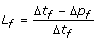with the added constraint that it monotonically decreases as the threshold decreases. Since neither the total number of edges nor the number of permuted edges are smooth functions, we approximate a smooth result by averaging finite differences over a range of widths. Figure 12 plots our estimated edge confidence as a function of the threshold for an instance of the ALARM network.

## INSURANCE network simulation study

To determine the generality of the above results we performed a similar study using the INSURANCE network. As was the case for the ALARM network, learning performance using DPSM improves as λ decreases, but in this case the best performance was obtained using BDe.

## Application to biological dataset

Figure 13 shows the consensus Bayesian network obtained by applying our method to the Glioblastoma dataset .

We use bagged gene shaving  to extract consensus gene clusters. Clusters containing genes with an obvious common biological function were also given a descriptive name. To generate a Bayesian network, we trichotomized the signed mean gene for each cluster into low, medium, and high values. We included additional nodes for clinical covariates of interest, including age, gender, whether chemotherapy or raditional was given and survival. We dichotomized survival into short and long survivors at 12 months, but marked as not available all censored survival times shorter than 12 months.

According to our analysis, survival is linked most closely with two clinical covariates, age and gender, and two gene clusters, a cluster of interferon induced genes and a cluster of growth inhibition genes.

## Conclusions and future work

We explored the space of model-averaging strategies in contexts with limited data with the goal of robustly learning large Bayesian networks. Our results follows.

1. 1.

Is bias-correction a good idea? In contexts with limited data, Bayesian bagging with scoring functions that permit the learning of overfitted models is a better strategy than using model-averaging with conservative scoring functions. In our experiments, bias-corrected scoring functions with Bayesian bagging have higher number of structural errors, both in terms of false positives (incorrect features) and false negatives (missing features).

2. 2.

Which is better: Bayesian bagging or ordinary bagging? Bayesian bagging yields models with more features than ordinary bagging. At high feature selection threshold values, Bayesian bagging yields better models, especially when sample sizes are small. As sample sizes increase, the two approaches are nearly indistinguishable in performance as measured by the number of false positives and false negatives. In limited data contexts, Bayesian bagging is superior to ordinary bagging.

3. 3.

How many bootstrap resamples are needed?: Our experiments show that for features whose true probability is close to the threshold for inclusion in the final model, up to 2500 bootstrap samples are needed for robust estimation. Previous studies [7, 10] have used one to two hundred bootstrap resamples. Noise features (false positives) are averaged out by bagging over such a large ensemble. This improvement comes not at the expense of false negatives, which are also reduced by the protocol.

4. 4.

Which class of scoring functions yield the most accurate models?: For the ALARM network, the DPSM scoring metric with small values of λ performed best, whereas for the INSURANCE network the BDe metric performed best. What both of these metrics have in common is that they very readily include additional edges into the network. A promiscuous scoring metric that produces overly complex models works well in the context of bagging because it is more likely to include true edges with weak support in each resample, hence significantly reducing false negatives, while the bagging method effectively eliminates the false positives introduced by the scoring metric.

5. 5.

How is a feature selection threshold to be determined?: We developed a permutation based method to compute significance thresholds for feature selection in bagged graphs. In the absence of a true model, the threshold gives us a bound on the number of false positive features in the final graph.

6. 6.

How many data samples are needed?: Dataset size is a key determiner of performance of the learning methods. Our model averaging strategy with bayesian bagging, and the DPSM scoring function (λ = 0.1) with feature selection using a threshold estimated by permutation testing, outperforms existing methods and yields excellent models even with samples sizes as low as 125 for the ALARM network. This result suggests that our model averaging approach is suitable for biological applications such as learning regulatory genetic networks, characterized by several tens of nodes and training data sets of the order of a few hundred samples.

One of the open questions is a theoretical characterization of the relationship between model complexity and sample size for Bayesian networks. In this paper, we characterized this relationship empirically for two well-known networks of moderate complexity. Our model averaging strategy learns more accurate models than other approaches when the amount of data is limited relative to the complexity of the model being learned. In future work we plan to explore more networks in biological applications, and refine our protocol for learning high confidence models with limited data.

## Abbreviations

AIC:

Aikake Information Criterion

BDe:

Bayesian Dirichlet metric

BIC:

Bayesian Information Criterion

DPSM:

Dirichlet Prior Scoring Metric

GBM:

Glioblastoma Multiforme

MCMC:

Markov Chain Monte Carlo

MDL:

Minimum Description Length

TCGA:

The Cancer Genome Atlas

UPSM:

Uniform Prior Scoring Metric.

## References

1. Heckerman D, Geiger D, Chickering DM: Learning Bayesian networks: the combination of knowledge and data. Machine Learning 1995, 20(3):197–243.

2. Buntine W: A guide to the literature on learning probabilistic networks from data. IEEE Transactions on Knowledge And Data Engineering 1996, 8: 195–210. 10.1109/69.494161

3. Cooper GF, Herskovits E: A Bayesian Method for Constructing Bayesian Belief Networks for Databases. In 7th Conference on Uncertainty in Artificial Intelligence. Edited by: D’Ambrosio BD, Smets P, Bonissone PP. Morgan Kaufmann; 1991:86–94.

4. Friedman N, Nachman I, Pe’er D: Learning Bayesian Network Structure from Massive Datasets: the ”Sparse Candidate” Algorithm. In Proc. Fifteenth Conference on Uncertainty in Artificial Intelligence (UAI ‘99). Edited by: Dubios H, Laskey K. Morgan Kaufmann; 1999:206–215.

5. Sachs K, Perez O, Pe’er D, Lauffenburger DA, Nolan GP: Causal Protein-Signaling Networks Derived from Multiparameter Single-Cell Data. Science 2005, 308(5721):523–529. 10.1126/science.1105809

6. Mukherjee S, Speed TP: Markov Chain Monte Carlo for Structural Inference with Prior Information. In Tech. Rep. 729. Department of Statistics, University of California Berkeley; 2007.

7. Friedman N, Goldszmidt M, Wyner A: Data Analysis with Bayesian Networks: A Bootstrap Approach. Proceedings of the 15th Conference on Uncertainty in Artificial Intelligence (UAI) 1999, 196–205.

8. Rodin AS, Boerwinkle E: Mining genetic epidemiology data with Bayesian networks I: Bayesian networks and example application (plasma apoE levels). Bioinformatics 2005, 21(15):3273–3278. 10.1093/bioinformatics/bti505

9. Efron B, Tibshirani R: An introduction to the bootstrap. Chapman and Hall, CRC Press; 1993.

10. Steck H, Jaakkola TS: Bias-Corrected Bootstrap and Model Uncertainty. In Advances in Neural Information Processing Systems 16. Edited by: Thrun S, Saul L, Schölkopf B. Cambridge, MA: MIT Press; 2004.

11. Rubin DB: The Bayesian Bootstrap. The Annals of Statistics 1981, 9: 130–134. 10.1214/aos/1176345338

12. Hastie T, Tibshirani R, Friedman J: The elements of statistical learning. In Springer Series on Statistics. Springer Verlag; 2001.

13. Friedman N, Koller D: Being Bayesian about Network Structure: A Bayesian Approach to Structure Discovery in Bayesian Networks. Machine Learning 2003, 50: 95–126. 10.1023/A:1020249912095

14. Madigan D, York J: Bayesian graphical models for discrete data. International Statistical Review 1995, 63: 215–232. 10.2307/1403615

15. Giudici P, Green P, Tarantola C: Efficient model determination for discrete graphical models. Biometrika 2000, 86: 785–801.

16. Yu J, Smith V, Wang P, Hartemink A, Jarvis E: Advances to Bayesian Network Inference for Generating Causal Networks from Observational Biological Data. Bioinformatics 2004, 20: 3594–3603. 10.1093/bioinformatics/bth448

17. Cooper GF, Herskovits E: A Bayesian method for the induction of probabilistic networks from data. Machine Learning 1992, 7: 299–347.

18. Yang S, Chang KC: Comparison of score metrics for Bayesian network learning. IEEE Transactions on Systems, Man and Cybernetics: Part A: Systems and Humans 2002, 32(3):419–428. 10.1109/TSMCA.2002.803772

19. Heckerman D, geiger D: Learning Bayesian networks: a unification for discrete and gaussian domains. Proceedings of the 11th Conference on Uncertainty in Artificial Intelligence 1995, 274–284.

20. Ripley BD: Pattern recognition and neural networks. MIT Press; 1996.

21. Chickering DM: Learning Bayesian networks is NP-complete. Learning from data: Artificial Intelligence and Statistics V 1996, 121–130.

22. Network TCGAR: Comprehensive genomic characterization defines human glioblastoma genes and core pathways. Nature 2008, 455(7216):1061–1068. [http://dx.doi.org/10.1038/nature07385] 10.1038/nature07385

23. Broom BM, Sulman EP, Do KA, Edgerton ME, Aldape KD: Bagged gene shaving for the robust clustering of high-throughput data. IJBRA 2010, 326–343.

## Acknowledgements

This research was supported in part by NIH/NCI grant R01 CA133996 and the Gulf Coast Center for Computational Cancer Research.

This article has been published as part of BMC Bioinformatics Volume 13 Supplement 13, 2012: Selected articles from The 8th Annual Biotechnology and Bioinformatics Symposium (BIOT-2011). The full contents of the supplement are available online at http://www.biomedcentral.com/1471-2105/13/S13/S1

## Author information

Authors

### Competing interests

The authors declare that they have no competing interests.

### Authors' contributions

BB and DS conceived of the study, and participated in its design and coordination and helped to draft the manuscript. KA consulted on statistical considerations. All authors read and approved the final manuscript.

## Rights and permissions

Reprints and Permissions